### Problems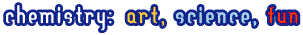### Best tasks from the past

In this section we publish best tasks from the previous IChO in Moscow held in 1996. Solution follows text of the problem with a two weeks gap. The tasks will be changed once a month. Have a nice time!Word

## Moscow, 1996. Physical chemistry

In 1908 E.Rutherford together with H.Geiger measured the rate ( x ) of emission of a -particles by radium (in the nature this element is represented by a single nuclide 226 Ra) and found that 1.00 g of radium emits x = 3.42 * 10 10 a -particles per second.

In 1911 Rutherford and American physical chemist B.Boltwood measured the rate of formation of helium from radium. This experiment permitted to obtain the most accurate value of Avogadro's number available at that time, given that the value of molar volume of ideal gas was well established. To achieve this goal a sample of radium salt purified from decay products and containing m = 192 mg of Ra was put into a device and the volume of the evolved helium was measured.

After 83 days ( t = 83.0 days) of the experiment 6.58 mm 3 of He was collected ( V He = 6.58 mm 3 corrected to 0 o C and 1 atm). To understand the results of this experiment we shall need the kinetic scheme of radioactive decay of Ra which is given below (half-lives are over the arrow, the type of decay is below the arrows):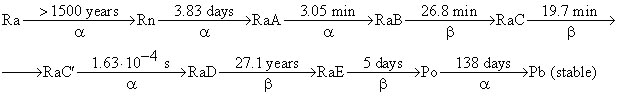(RaA - RaE are intermediate products of radon decay).

1. Write down the first six radioactive decays using a modern notation showing atomic and mass numbers of all nuclei involved.

In a rough approximation, half-lives of all radium decay products, except those of RaD and Po, may be assumed to be negligible compared to the time of measurement, t . Using this approximation perform the following calculations:

2. a) How many helium atoms were formed from each decayed radium atom after 83 days"

b) How many helium atoms were formed in total during the experiment"

3. Calculate an approximate value of Avogadro's number from the above data.

For a more accurate computation of Avogadro's number the half-life of radon T 1/2 (Rn) = 3.83 days cannot be neglected as it is comparable with the duration of experiment t and not all of radon atoms decayed to the end of experiment.

4. Choose which of the plots given below displays the time dependence of the number of radon atoms N Rn in the course of the experiment.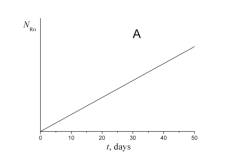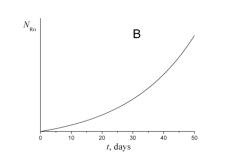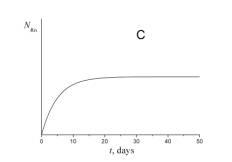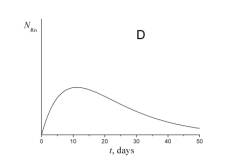5. Choose which of the plots given below shows the time dependence of the volume of helium V He of radon atoms in the course of the experiment.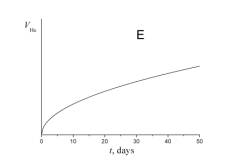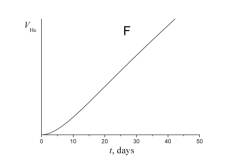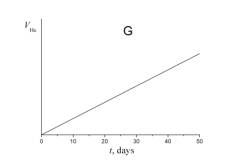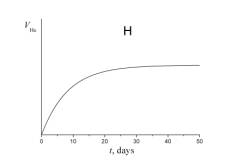6. a) Using a short kinetic schemeand the plot which you have selected in question 4, write a relation between the number of radon atoms at the end of experiment N ¢ Rn and the number of radium atoms N Ra .

b) Evaluate N ¢ Rn using the rate of radium decay given above ( x = 3.42 * 10 10 a -particles per gram of radium per second).

7. How many helium atoms could be formed from radon atoms remaining at the end of experiment N ¢ Rn if all these atoms had decayed to RaD"

8. Using your answers to the above questions calculate a better approximation to: a) the number of helium atoms formed; b) the Avogadro's number.

## Moscow, 1996. Inorganic chemistryMoscow, 1996. Organic chemistry

| ©2006-2007 Chemisty Department of Moscow State University |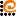© web-design www.AdCode.ru